# Class equation of a group relative to a prime power

## Statement

Suppose$G$ is a finite group and$p$ is a prime. Suppose$p^k$ is a power of$p$ that divides the order of$G$. Let$S$ be the subgroup of the center of$G$ comprising those elements whose order is relatively prime to$p$. Further, for any element$x \in G$, denote$a(x,n)$ the number of solutions to$g^n = x$. Similarly, for any subset$B$ of$G$, let$a(B,n)$ be the number of solutions to$g^n \in B$.

We have the following three facts:

•$a(s,p^k) = a(e,p^k)$ for all$s \in S$.
•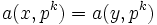$a(x,p^k) = a(y,p^k)$ for any$x,y$ that are conjugate in$G$, so$a(c,p^k) = |c|a(x,p^k)$ where$c$ is a conjugacy class and$x \in c$.
•$|G| = |S|a(e,p^k) + \sum_c a(c,p^k) = |S|a(e,p^k) + \sum_c [G:C_G(x)]a(x,p^k)$

where$e$ is the identity element and$c$ varies over all conjugacy classes of$G$ not contained in$S$, and$x$.

## Facts used

1. kth power map is bijective iff k is relatively prime to the order
2. Cauchy's theorem for abelian groups: If$p$ divides the order of a group, the group has an element of order$p$.
3. Size of conjugacy class equals index of centralizer

## Proof

The proof is essentially a counting argument. The right side partitions the elements of$G$ according to the conjugacy class where their$(p^k)^{th}$ power resides. For simplicity of notation, we let$n = p^k$.

### Elements for which the power is in the subgroup$S$

Claim: For$s \in S$, we have$a(s,n) = a(e,n)$.

Proof:

1. The order of$S$ is relatively prime to$p$, and hence, relatively prime to$n$: Since the order of every element of$S$ is relatively prime to$p$, fact (2) yields that the order of$S$ is relatively prime to$p$.
2. For every element$s \in S$, there exists$g \in S$ such that$g^n = s$: This follows from fact (1), and the construction of$S$ as a subgroup of order relatively prime to the center.
3. The map$x \mapsto gx$ is a bijection between the set of solutions to$x^n = e$ and the set of solutions to$x^n = s$: If$x^n = e$, then$(gx)^n = g^nx^n = sx^n = s$ (note that we use that$S$ is in the center to write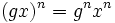$(gx)^n = g^nx^n$). Further, if$(gx)^n = s$, then$x^n = e$ by the same argument. Since left multiplication is bijective on$G$, we obtain that$x \mapsto gx$ is a bijection.
4.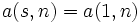$a(s,n) = a(1,n)$ for all$s \in S$: This follows by taking cardinalities on the preceding step.

The claim yields that the total number of elements whose$n^{th}$ power is in$S$ is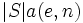$|S|a(e,n)$.

### Elements for which the power is outside the subgroup

Claim: If$gxg^{-1} = y$, then$a(x,n) = a(y,n)$.

Proof: This follows from the fact that conjugation by$g$ establishes a bijection between$n^{th}$ roots of$x$ and$n^{th}$ roots of$y$. In other words: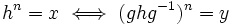$h^n = x \iff (ghg^{-1})^n = y$.

Thus, the total number of elements whose$n^{th}$ power is in the conjugacy class of$x$ equals the product of the size of the conjugacy class and$a(x,n)$. Fact (3) now yields that the total number of elements is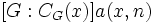$[G:C_G(x)]a(x,n)$.

### Summing up

Summing up over all elements of$G$ based on where their$n^{th}$ powers fall gives this formula.